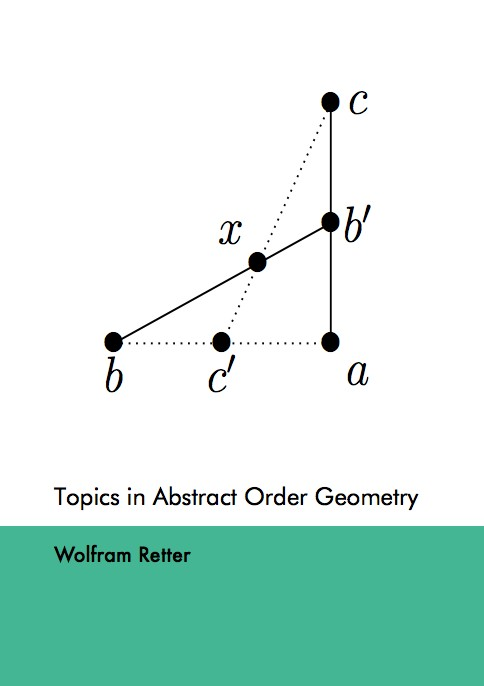Datenbestand vom 13. August 2019aktualisiert am 13. August 2019

# ISBN 9783843912556Euro 84,00 inkl. 7% MwSt

978-3-8439-1255-6, Reihe Mathematik

Wolfram Retter
Topics in Abstract Order Geometry

185 Seiten, Dissertation Technische Universität Hamburg-Harburg (2013), Softcover, B5

## Zusammenfassung / Abstract

Abstract order geoemetry investigates geometric interval spaces - sets with a geometric interval relation, i.e. a ternary relation satisfying some axioms supporting the interpretation that three points are related iff looking from the first one, the second one is in front of the third one. A metric space becomes a geometric interval space when defining this relation to hold iff the distance from the first to the third point is the sum of the other two distances.

Three of the axioms for a geometric interval relation say: Looking from any point, the binary relation ’is in front of’ is reflexive, transitive, antisymmetric. The relation ’is in front of’ looking from a convex set is investigated. The following conditions on that relation are equivalent to other axioms of order geometry: Transitivity, looking from any convex set, and antisymmetry, looking from any convex set and only looking outside that set. The symmetrized relation ’is in line with’, looking from any point and only looking outside that point, is also proved to be equivalent to two further axioms of order geometry.

The new concept of a topological interval space is defined, and it is proved: In a modular geometric topological interval space, each non-empty compact convex set is gated. A criterion is proved for an interval space to have an embedding into a median space. The neighbor-joining method for reconstructing a tree from the distances between its vertices of degrees one and two is embedded in the context of abstract order geometry.

A metric space may have non-isometric median closures. The new concept of a quadrimedian interval space, intermediate between a median and an arboric interval space (i.e. treelike structure), is defined, and it is proved that any two quadrimedian metric spaces that are median closures of a metric space are isometric, in analogy to the theorem of Steinitz on algebraic closures of a field. The new concept of a median-extremal point is defined, and for a compact quadrimedian geometric topological interval space it is proved that it is the convex closure of its median-extremal points, in analogy to the Krein-Milman theorem for locally convex topological vector spaces. Thus, analogues of two central structure theorems of algebra and analysis are valid for quadrimedian spaces that are not valid for median spaces: The concept of a quadrimedian interval space seems to be a natural one. Two applications of quadrimedian spaces to arboric spaces are given.